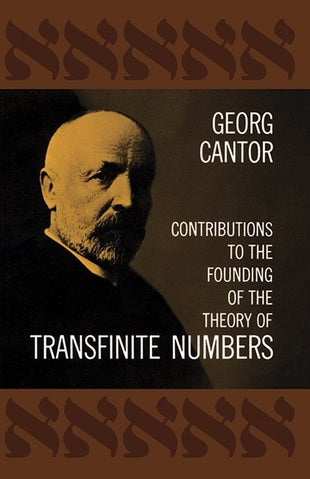# Contributions to the Founding of the Theory of Transfinite Numbers by Georg Cantor (Author)_____________________________

'Contributions to the Founding of the Theory of Transfinite Numbers'
By Georg Cantor (Author)

Purchase Book:

Amazon.com
Amazon.co.uk

Description:

One of the greatest mathematical classics of all time, this work established a new field of mathematics which was to be of incalculable importance in topology, number theory, analysis, theory of functions, etc., as well as in the entire field of modern logic. It is rare that a theory of such fundamental mathematical importance is expressed so simply and clearly: the reader with a good grasp of college mathematics will be able to understand most of the basic ideas and many of the proofs. Cantor first develops the elementary definitions and operations of cardinal and ordinal numbers and analyzes the concepts of "canlinality" and "ordinality." He covers such topics as the addition, multiplication, and exponentiation of cardinal numbers, the smallest transfinite cardinal number, the ordinal types of simply ordered aggregates, operations on ordinal types, the ordinal type of the linear continuum, and others. He then develops a theory of well-ordered aggregates, and investigates the ordinal numbers of well-ordered aggregates and the properties and extent of the transfinite ordinal numbers.

An 82-page introduction by the eminent mathematical historian Philip E. B. Jourdain first sketches the background of Cantor's theory, discussing the contributions of such predecessors as Veicrstrass, Cauchy, Dedekind, Dirichlet, Riemann, Fourier, and Hankel; it then traces the development of the theory by summarizing and analyzing Cantor's earlier work. A bibliographical note provides information on further investigations in the theory of transfinite numbers by Frege, Peano, Whitehead, Russell, etc.

"Would serve as well as any modern textto initiate a student in this exciting branch of mathematics."

(
Mathematical Gazette)

________________________________________________

Georg Ferdinand Ludwig Philipp Cantor born March 3 [O.S. February 19] 1845 – January 6, 1918) was a German mathematician. He invented set theory, which has become a fundamental theory in mathematics. Cantor established the importance of one-to-one correspondence between the members of two sets, defined infinite and well-ordered sets, and proved that the real numbers are more numerous than the natural numbers. In fact, Cantor's method of proof of this theorem implies the existence of an "infinity of infinities". He defined the cardinal and ordinal numbers and their arithmetic. Cantor's work is of great philosophical interest, a fact of which he was well aware.

_____________________________

_____________________________